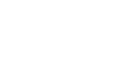Railo 4.2 Reference
Choose a function:

## Function RANDRANGE

Generates a random integer between two specified numbers.
Requests for random integers that are greater than 100,000,000
result in non-random numbers, to prevent overflow during
internal computations.

## Example

`randrange(number number1,number number2,[string algorithm]):number`

## Arguments

The arguments for this function are set. You can not use other arguments except the following ones.
Name Type Required Description
number1 number  Yes smaller number
number2 number  Yes bigger number
algorithm string  No The algorithm to use to generate the random number.
- CFMX_COMPAT (very simple and not very secure algorithm (default)).
- SHA1PRNG (generates a number using the Sun Java SHA1PRNG algorithm. This algorithm provides greater randomness than the default algorithm)
- IBMSecureRandom (IBM JVM does not support the SHA1PRNG algorithm)